Скачать презентацию Frugal Path Mechanisms by Aaron Archer and Eva

440c99ff3fea82b3b854a6ea9f2e7da1.ppt

• Количество слайдов: 17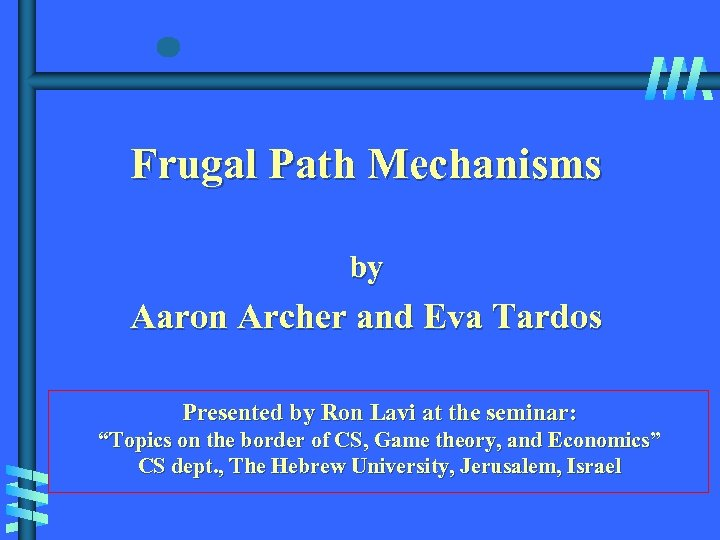Frugal Path Mechanisms by Aaron Archer and Eva Tardos Presented by Ron Lavi at the seminar: “Topics on the border of CS, Game theory, and Economics” CS dept. , The Hebrew University, Jerusalem, Israel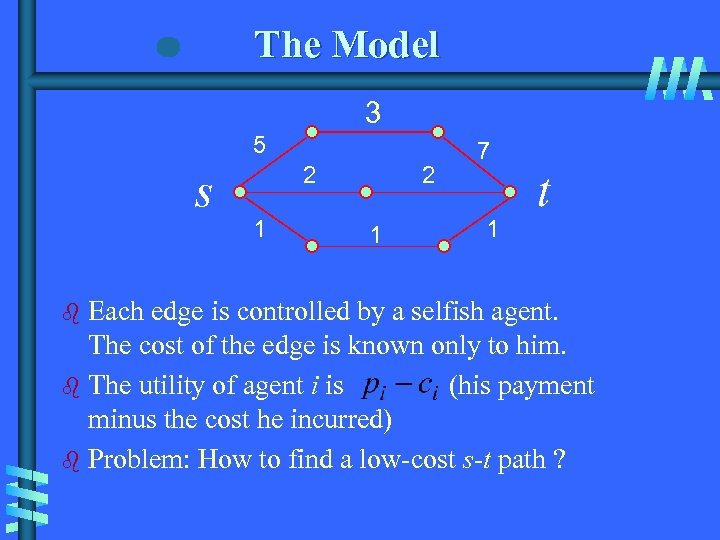The Model 3 5 s 2 1 7 1 t Each edge is controlled by a selfish agent. The cost of the edge is known only to him. b The utility of agent i is (his payment minus the cost he incurred) b Problem: How to find a low-cost s-t path ? b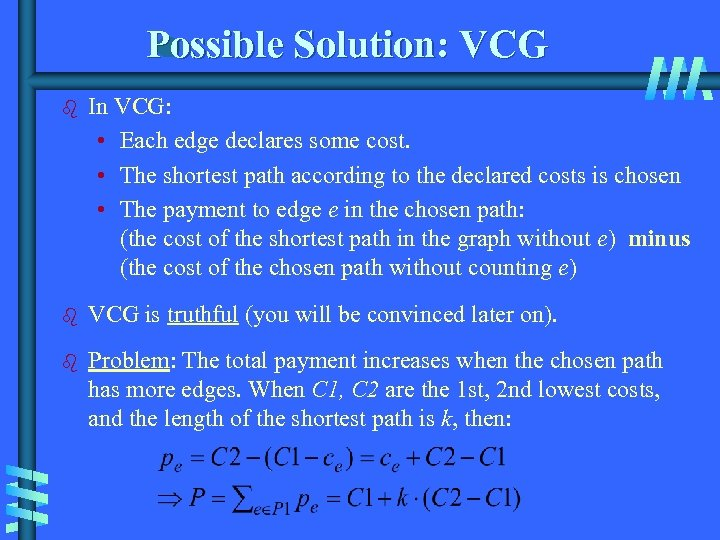Possible Solution: VCG b In VCG: • Each edge declares some cost. • The shortest path according to the declared costs is chosen • The payment to edge e in the chosen path: (the cost of the shortest path in the graph without e) minus (the cost of the chosen path without counting e) b VCG is truthful (you will be convinced later on). b Problem: The total payment increases when the chosen path has more edges. When C 1, C 2 are the 1 st, 2 nd lowest costs, and the length of the shortest path is k, then: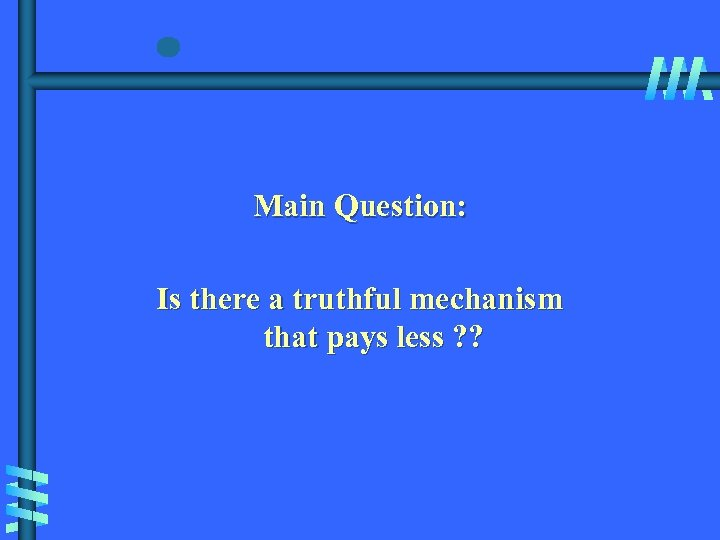Main Question: Is there a truthful mechanism that pays less ? ?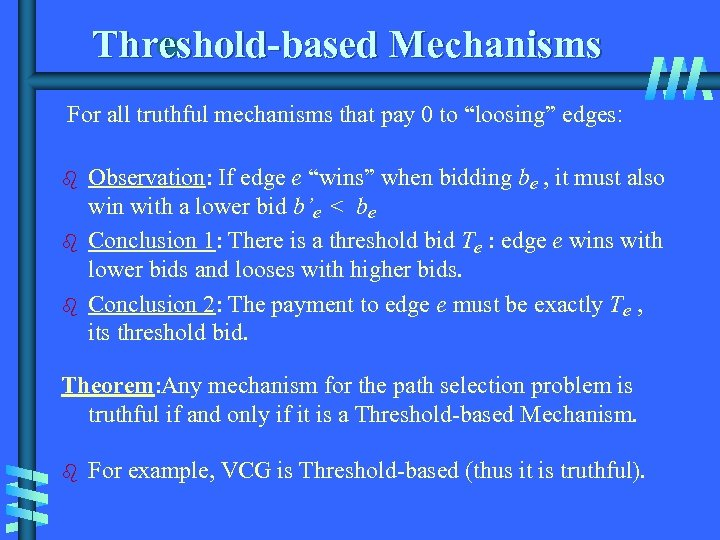Threshold-based Mechanisms For all truthful mechanisms that pay 0 to “loosing” edges: b b b Observation: If edge e “wins” when bidding be , it must also win with a lower bid b’e < be Conclusion 1: There is a threshold bid Te : edge e wins with lower bids and looses with higher bids. Conclusion 2: The payment to edge e must be exactly Te , its threshold bid. Theorem: Any mechanism for the path selection problem is truthful if and only if it is a Threshold-based Mechanism. b For example, VCG is Threshold-based (thus it is truthful).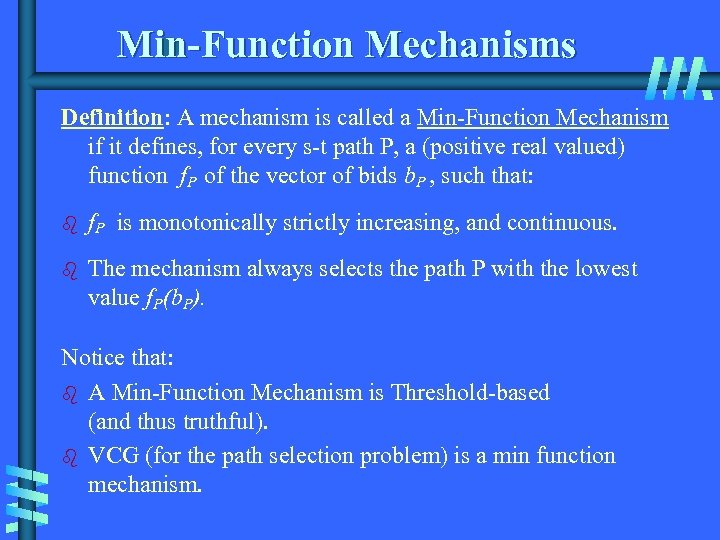Min-Function Mechanisms Definition: A mechanism is called a Min-Function Mechanism if it defines, for every s-t path P, a (positive real valued) function f. P of the vector of bids b. P , such that: b f. P b is monotonically strictly increasing, and continuous. The mechanism always selects the path P with the lowest value f. P(b. P). Notice that: b A Min-Function Mechanism is Threshold-based (and thus truthful). b VCG (for the path selection problem) is a min function mechanism.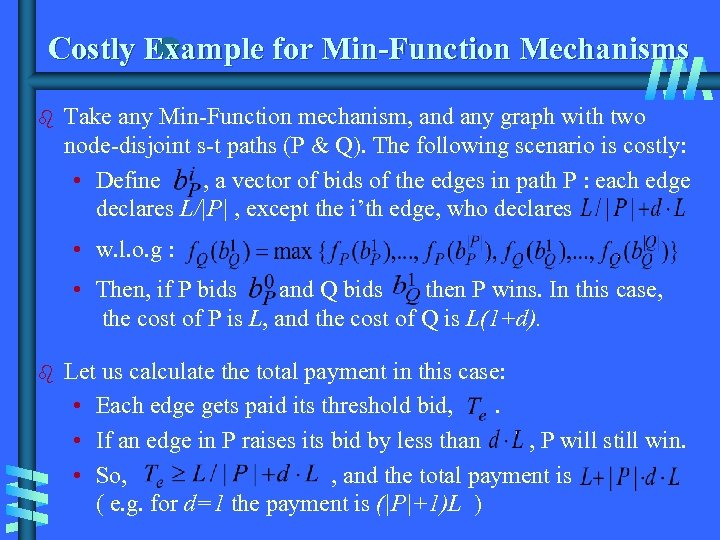Costly Example for Min-Function Mechanisms b Take any Min-Function mechanism, and any graph with two node-disjoint s-t paths (P & Q). The following scenario is costly: • Define , a vector of bids of the edges in path P : each edge declares L/|P| , except the i’th edge, who declares • w. l. o. g : • Then, if P bids and Q bids then P wins. In this case, the cost of P is L, and the cost of Q is L(1+d). b Let us calculate the total payment in this case: • Each edge gets paid its threshold bid, . • If an edge in P raises its bid by less than , P will still win. • So, , and the total payment is ( e. g. for d=1 the payment is (|P|+1)L )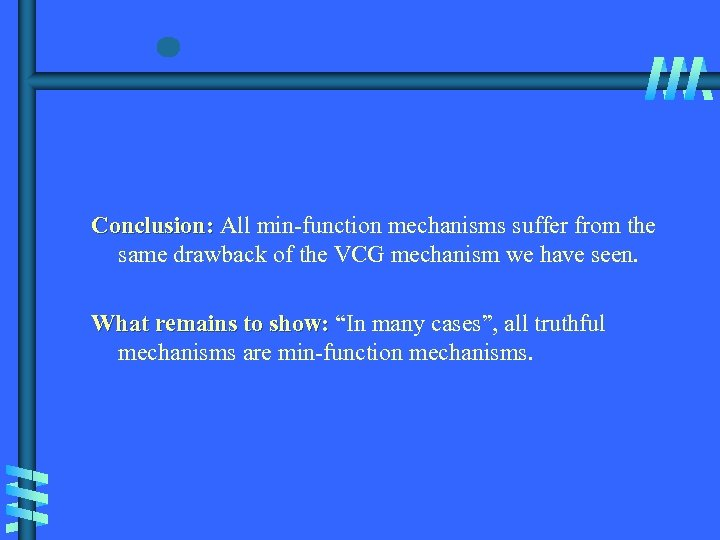Conclusion: All min-function mechanisms suffer from the same drawback of the VCG mechanism we have seen. What remains to show: “In many cases”, all truthful mechanisms are min-function mechanisms.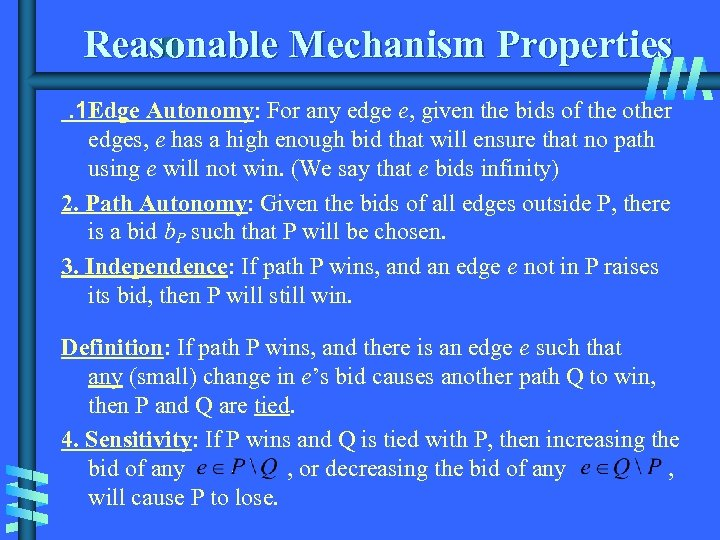Reasonable Mechanism Properties. 1 Edge Autonomy: For any edge e, given the bids of the other edges, e has a high enough bid that will ensure that no path using e will not win. (We say that e bids infinity) 2. Path Autonomy: Given the bids of all edges outside P, there is a bid b. P such that P will be chosen. 3. Independence: If path P wins, and an edge e not in P raises its bid, then P will still win. Definition: If path P wins, and there is an edge e such that any (small) change in e’s bid causes another path Q to win, then P and Q are tied. 4. Sensitivity: If P wins and Q is tied with P, then increasing the bid of any , or decreasing the bid of any , will cause P to lose.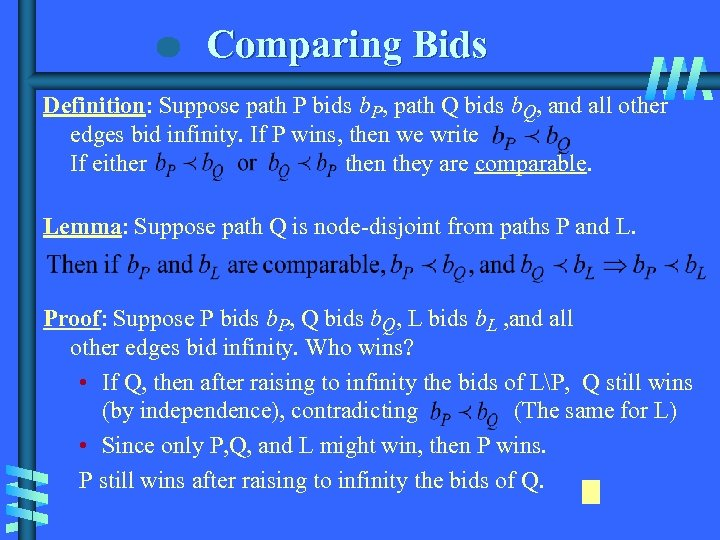Comparing Bids Definition: Suppose path P bids b. P, path Q bids b. Q, and all other edges bid infinity. If P wins, then we write If either then they are comparable. Lemma: Suppose path Q is node-disjoint from paths P and L. Proof: Suppose P bids b. P, Q bids b. Q, L bids b. L , and all other edges bid infinity. Who wins? • If Q, then after raising to infinity the bids of LP, Q still wins (by independence), contradicting (The same for L) • Since only P, Q, and L might win, then P wins. P still wins after raising to infinity the bids of Q.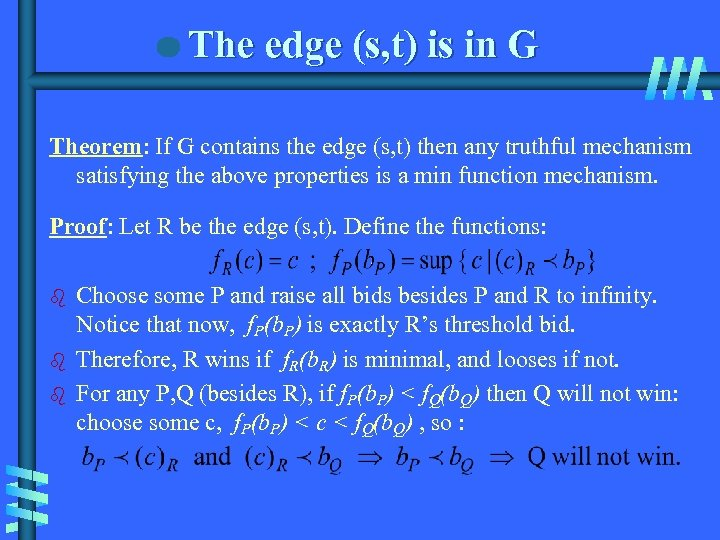The edge (s, t) is in G Theorem: If G contains the edge (s, t) then any truthful mechanism satisfying the above properties is a min function mechanism. Proof: Let R be the edge (s, t). Define the functions: b b b Choose some P and raise all bids besides P and R to infinity. Notice that now, f. P(b. P) is exactly R’s threshold bid. Therefore, R wins if f. R(b. R) is minimal, and looses if not. For any P, Q (besides R), if f. P(b. P) < f. Q(b. Q) then Q will not win: choose some c, f. P(b. P) < c < f. Q(b. Q) , so :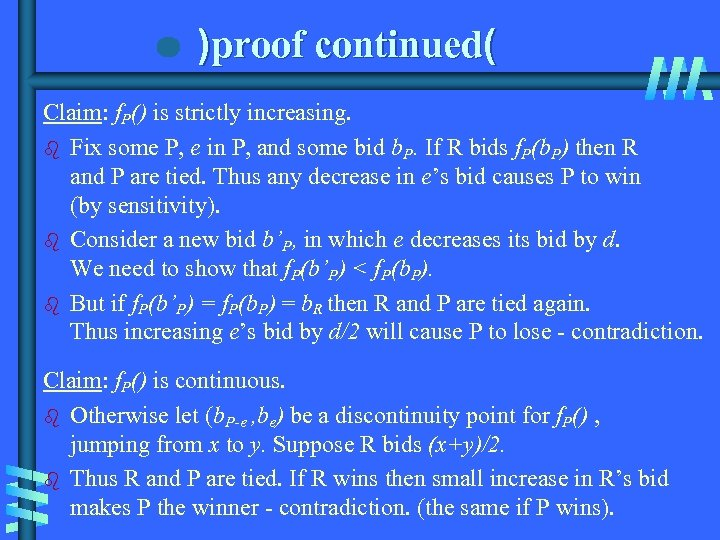)proof continued( Claim: f. P() is strictly increasing. b Fix some P, e in P, and some bid b. P. If R bids f. P(b. P) then R and P are tied. Thus any decrease in e’s bid causes P to win (by sensitivity). b Consider a new bid b’P, in which e decreases its bid by d. We need to show that f. P(b’P) < f. P(b. P). b But if f. P(b’P) = f. P(b. P) = b. R then R and P are tied again. Thus increasing e’s bid by d/2 will cause P to lose - contradiction. Claim: f. P() is continuous. b Otherwise let (b. P-e , be) be a discontinuity point for f. P() , jumping from x to y. Suppose R bids (x+y)/2. b Thus R and P are tied. If R wins then small increase in R’s bid makes P the winner - contradiction. (the same if P wins).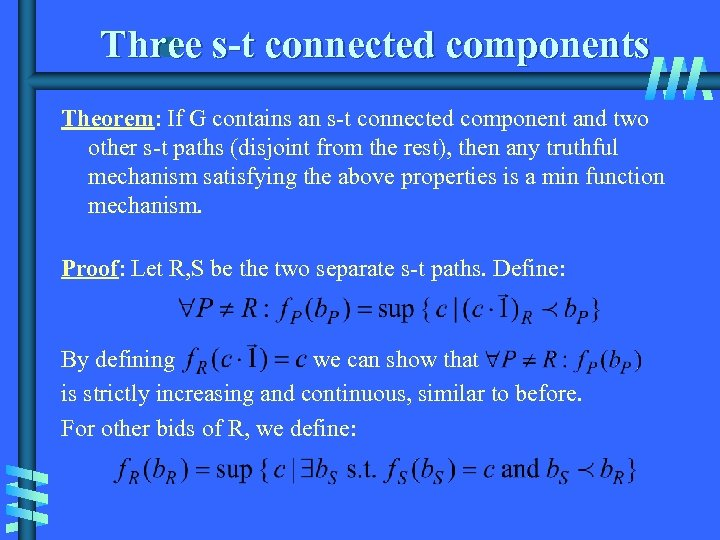Three s-t connected components Theorem: If G contains an s-t connected component and two other s-t paths (disjoint from the rest), then any truthful mechanism satisfying the above properties is a min function mechanism. Proof: Let R, S be the two separate s-t paths. Define: By defining we can show that is strictly increasing and continuous, similar to before. For other bids of R, we define: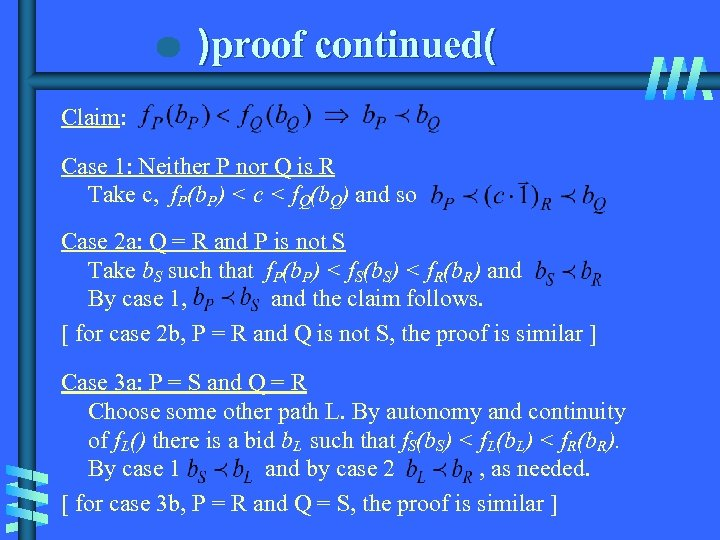)proof continued( Claim: Case 1: Neither P nor Q is R Take c, f. P(b. P) < c < f. Q(b. Q) and so Case 2 a: Q = R and P is not S Take b. S such that f. P(b. P) < f. S(b. S) < f. R(b. R) and By case 1, and the claim follows. [ for case 2 b, P = R and Q is not S, the proof is similar ] Case 3 a: P = S and Q = R Choose some other path L. By autonomy and continuity of f. L() there is a bid b. L such that f. S(b. S) < f. L(b. L) < f. R(b. R). By case 1 and by case 2 , as needed. [ for case 3 b, P = R and Q = S, the proof is similar ]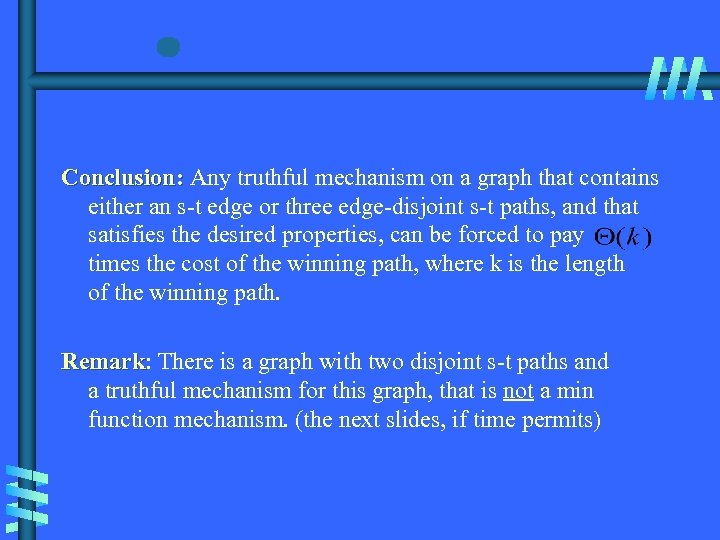Conclusion: Any truthful mechanism on a graph that contains either an s-t edge or three edge-disjoint s-t paths, and that satisfies the desired properties, can be forced to pay times the cost of the winning path, where k is the length of the winning path. Remark: There is a graph with two disjoint s-t paths and Remark a truthful mechanism for this graph, that is not a min function mechanism. (the next slides, if time permits)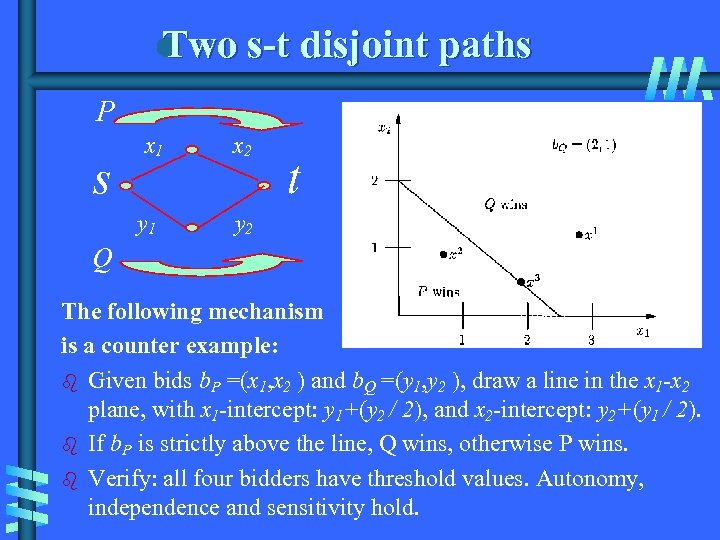Two s-t disjoint paths P s x 1 y 1 x 2 t y 2 Q The following mechanism is a counter example: b Given bids b. P =(x 1, x 2 ) and b. Q =(y 1, y 2 ), draw a line in the x 1 -x 2 plane, with x 1 -intercept: y 1+(y 2 / 2), and x 2 -intercept: y 2+(y 1 / 2). b If b. P is strictly above the line, Q wins, otherwise P wins. b Verify: all four bidders have threshold values. Autonomy, independence and sensitivity hold.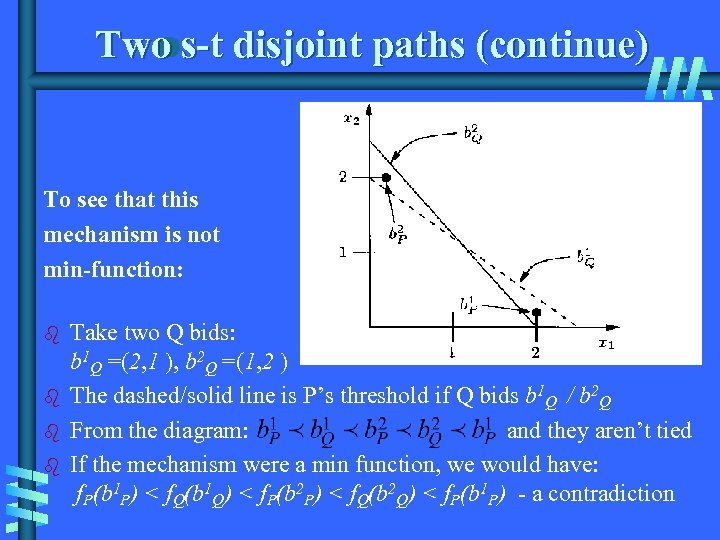Two s-t disjoint paths (continue) To see that this mechanism is not min-function: b b Take two Q bids: b 1 Q =(2, 1 ), b 2 Q =(1, 2 ) The dashed/solid line is P’s threshold if Q bids b 1 Q / b 2 Q From the diagram: and they aren’t tied If the mechanism were a min function, we would have: f. P(b 1 P) < f. Q(b 1 Q) < f. P(b 2 P) < f. Q(b 2 Q) < f. P(b 1 P) - a contradiction